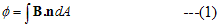# Magnetic Flux

## Magnetic Flux

• The flux of magnetic field through a surface is defined in a similar manner as we defined flux in the electric field
• if dA be an area element on any arbitrary surface and n be the unit vector perpendicular to the area element then magnetic flux is defined as• If the surface under considerations is a plane of area A and magnetic field is constant in both the magnitude and direction over the surface and θ is the angle between magnetic field B and unit normal to the surface then magnetic flux is given by
Φ=BAcosθ                                  --(2)
• Unit of flux is Wb(weber)
1Wb=1T.m2